﻿ Lecture 34 Summary
• Diffusion
• Photoelectric effect
• Quiz bonus diffusion

•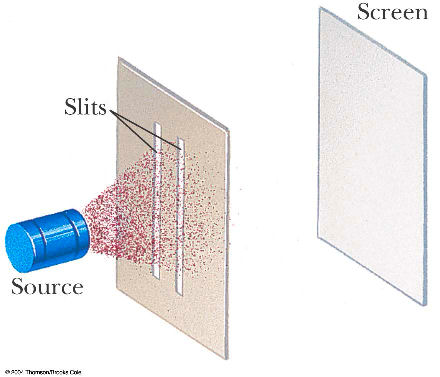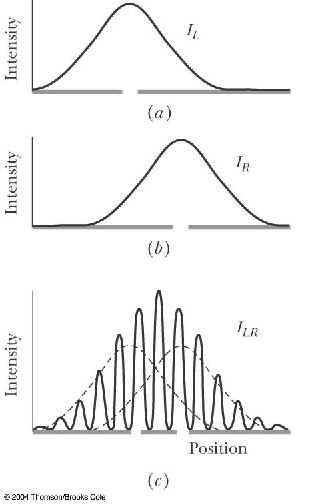• The de Broglie hypothesis
•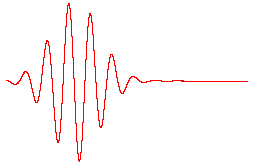• wave-particle duality video

•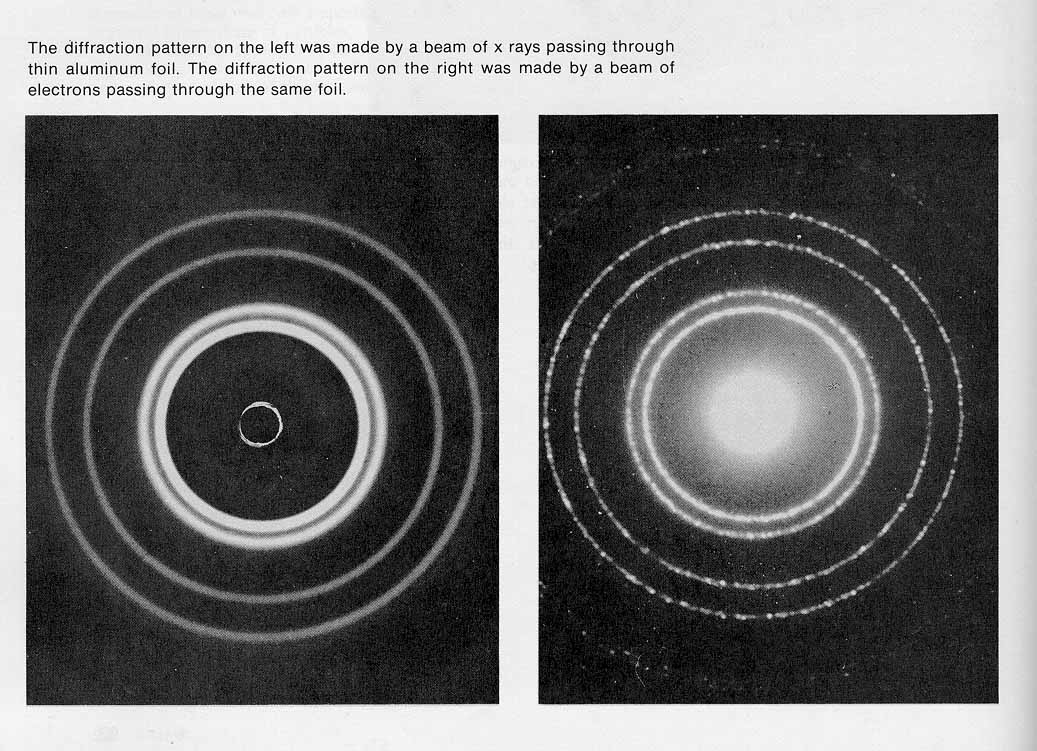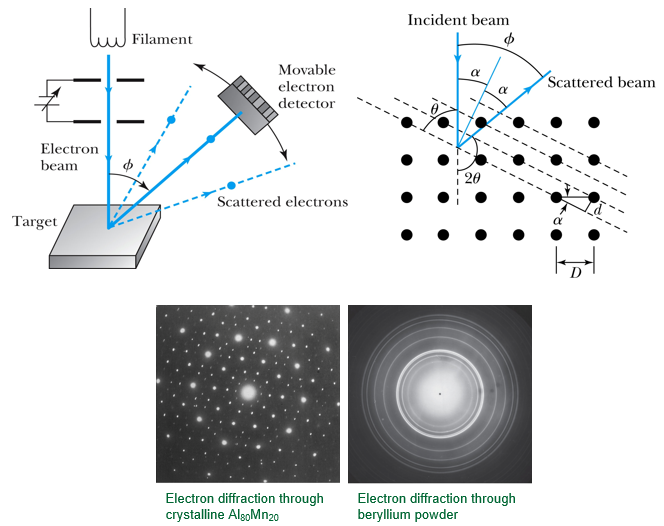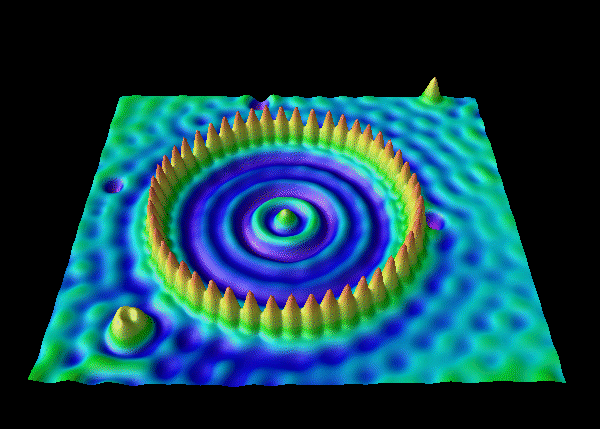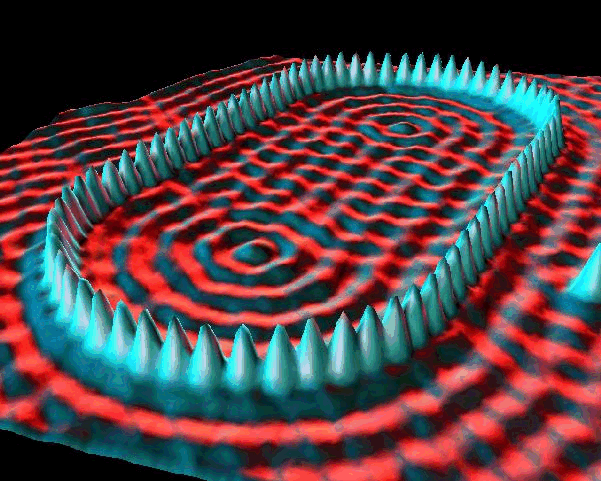• Lecture learning outcomes
A student who masters the topics in this lecture will be able to:
• describe how particles (like electrons and protons) can have wavelike properties, such as showing interference and diffraction patterns
• use algebra to find the de Broglie wavelength λ or momentum p of a particle when either one of these quantities is given

• Practice:
• Example #2

Example #3

• Prepare:
Read textbook sections 31-1 through 31-4 before the next lecture

Ball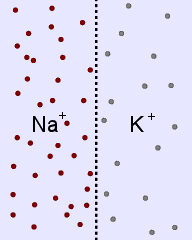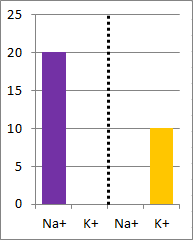Sodium and potassium ions in solution occupy identical volumes that are separated by a removable barrier. The concentration of Na+ is 20 mmol/L on the left side, and there is 10 mmol/L of K+ on the right side. The diffusion coefficients for each ion are the same. There is a picture of the initial conditions above, as well as a bar graph showing the initial concentrations on each side of the container. Which of the following bar graphs show the concentrations a short time after the barrier is removed?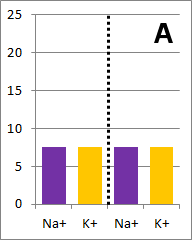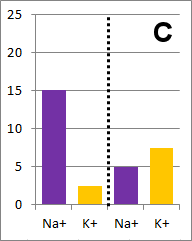kw5
If an electron and a proton have the same speed, which would have the longer wavelength?
A. The electron.
B. The proton.
C. They would have the same wavelength.

Walker5e EYU 30.5
The de Broglie waves for two particles of equal mass are shown below. The kinetic energy of particle 1 is _____ the kinetic energy of particle 2.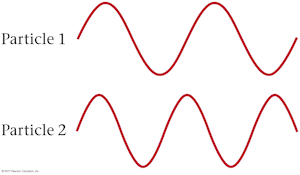A. greater than
B. less than
C. equal to

Walker5e 30.69
A researcher uses laser cooling to build an atomic trap that contains rubidium atoms of mass 1.42×10−25 kg. If the atoms are separated by 75 nm on average, what speed should they have so that their de Broglie wavelengths are equal to their average separation?
A. 285 km/s
B. 706 m/s
C. 16.1 m/s
D. 0.0623 m/sThe diffusion rate for Na+ will be twice as fast as for K+ because its concentration gradient is twice as large. Bar graph B depicts the concentrations at equilibrium, after a long time (each ion uniformly distributed throughout the entire volume).

A. The electron.

The de Broglie hypothesis says the wavelength of a particle's matter wave is inversely proportional to its momentum. Therefore the smaller mass and smaller momentum of the electron means that it has a longer wavelength.

B. less than

The longer de Broglie wavelength of particle 1 means that it has less momentum than particle 2 because de Broglie wavlength is inversely proportional to momentum. Because the two particles have the same mass, this also means particle 1 has a smaller velocity and less kinetic energy than particle 2.

D. 0.0623 m/s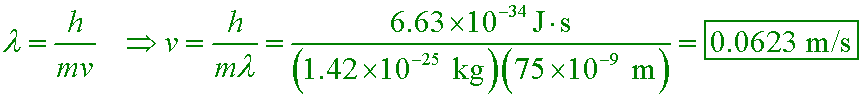The temperature of the atoms needs to be 20 µK if the most probable speed of the atoms is to be this slow! Only atom traps can achieve temperatures this low.
In general, overlapping de Broglie waves lead to quantum behavior. This is the basic technique used to produce a Bose-Einstein condensate, which is a quantum state of matter.